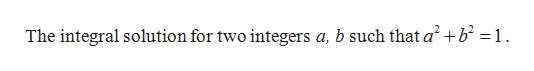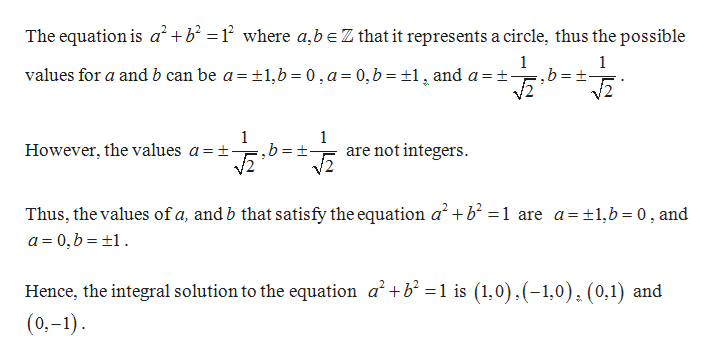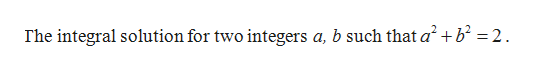Find all integral solutions (a, b) ∈ Z2 for each of the following three equations:a2+b2=1,a2+b2=2 and a2+b2=9.

Question

Find all integral solutions (a, b) ∈ Z2 for each of the following three equations:

a2+b2=1,a2+b2=2 and a2+b2=9.

Step 1
1. To find:help_outlineImage TranscriptioncloseThe integral solution for two integers a, b such that a2 +b =1 fullscreen
Step 2

Solution:help_outlineImage TranscriptioncloseThe equation is a2+b = where a,beZ that it represents a circle, thus the possible 1 1 values for a and b can be a and a = t 1,b 0,a= 0,b =1 1 1 are not integers However, the values a = t- Thus, the values of a, and b that satisfy the equation a a 0, b 1 +b = 1 are a=1,b 0, and Hence, the integral solution to the equation a2+b =1 is (1,0),(-1,0). (0,1) and (0,-1) _ fullscreen
Step 3

To find:...help_outlineImage TranscriptioncloseThe integral solution for two integers a, b such that a2 +b 2 fullscreen

Want to see the full answer?

See Solution

Want to see this answer and more?

Our solutions are written by experts, many with advanced degrees, and available 24/7

See Solution
Tagged in

Math